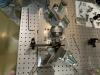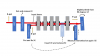Reports 1-1 of 1
IOO (OMC)
yohei.nishino - 21:47 Monday 16 May 2022 (20747) Print this report
OFI inspection

Aso, Michael, Nishino,

Here we report on the results of OFI inspection and its procedure. I attached a rough sketch of the procedure below.

## Procedure

1. We first maximized S-pol power with an HWP and polarizer and measured the transmissivity in the forward direction (T_f). (The polarizer was removed when measuring T_b)
2. we put a mirror* in front of the OFI's PBS to reflect back the transmitted beam to OFI. Here the mirror is slightly tilted from vertical incidence to separate the transmitted beam in the backward direction from the input beam.
3. In this setup, we cannot see the backward transmitted light by eyes, so we inserted an HWP in front of the QP (see figure), and slightly change the polarization angle to visualize the backward transmission.
4. Put and fix a power meter on the backward transmitted beam position
5. Remove the inserted HWP and record the value of the power meter (Backward transmitted power)
6. Put the power meter in front of the 2nd PBS and measure the forward transmitted power again.

*We measured its reflectivity beforehand (=95.4%).

## Result

T_f = (Forward transmitted power [mW]) / (Input power [mW]) = 1.662/1.965 = 97.3%

T_b = (Backward transmitted power [μW]) / (Forward transmitted power [mW]) = 143.0/26.32 = 0.54 %

Extinction ratio = 10*log10(T_f/T_b) = 22.5 [dB]

The designed value is 33 dB, so we need more investigation.

Images attached to this reportyohei.nishino - 22:05 Tuesday 17 May 2022 (20765) Print this report

## Result

T_f = (Forward transmitted power [mW]) / (Input power [mW]) = 1.662/1.965 = 97.3%

T_b = (Backward transmitted power [μW]) / (Forward transmitted power [mW]) = 143.0/26.32 = 0.54 %

Extinction ratio = 10*log10(T_f/T_b) = 22.522.6 [dB]

The designed value is 33 dB, so we need more investigation.

×

×